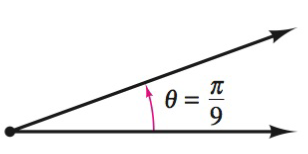Chapter 8.1, Problem 7E### Calculus: An Applied Approach (Min...

10th Edition
Ron Larson
ISBN: 9781305860919

#### Solutions

Chapter
Section### Calculus: An Applied Approach (Min...

10th Edition
Ron Larson
ISBN: 9781305860919
Textbook Problem
1 views

# Finding Conterminal Angles In Exercise 7-10, determine two conterminal angles in radian measure (one positive and one negative) for the angle.To determine

To calculate: The two coterminal angles in radian measure, in which one is positive and another is negative with the help of provided diagram,Explanation

Given Information:

The provided angle is θ=π9 radians, which is shown below as:

Formula used:

The angle measuring θ has same initial and terminal sides as the angle measuring 360θ, such angles are coterminal angle.

Calculation:

Draw diagram for coterminal angles and provided angle for θ=π2 radians.

From above diagram, let θ+ be the positive coterminal angle and θ be the negative coterminal angle

### Still sussing out bartleby?

Check out a sample textbook solution.

See a sample solution

#### The Solution to Your Study Problems

Bartleby provides explanations to thousands of textbook problems written by our experts, many with advanced degrees!

Get Started

#### Find the limit. lim0sin+tan

Single Variable Calculus: Early Transcendentals, Volume I

#### In Exercises 47-52, find and simplify f(a+h)f(a)h (h 0) for each function. 49. f(x) = x3 x

Applied Calculus for the Managerial, Life, and Social Sciences: A Brief Approach

#### Insert the proper sign to replacein problems 7-14. 14.

Mathematical Applications for the Management, Life, and Social Sciences

#### Write the following numbers in word form. 4.

Contemporary Mathematics for Business & Consumers

#### limx0cscx= a) 0 b) c) d) does not exist

Study Guide for Stewart's Single Variable Calculus: Early Transcendentals, 8th

#### The quadratic approximation for at a = 1 is:

Study Guide for Stewart's Multivariable Calculus, 8th